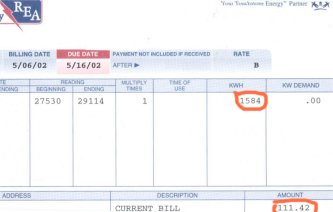Electricity cost:

```Electricity is sold by the kilowatt-hour (kWh).

Electric prices may vary from region to region
and can be seasonal as well.
```
``
How to determine the KWH price:
```The kWh price is found by dividing the total
monthly bill amount by the total kWh usage.

Example:  \$111.42 / 1584 kWh  = \$0.08 ```
```Enter the kWh price into the input box,
if different.

The Profit Potential Calculator will analyze and
calculate the total energy cost for using
the Genesis Series-V Aeroponic system.```
` `